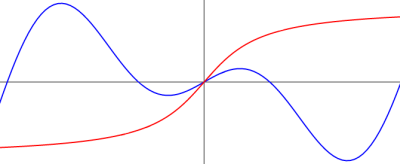# Experiments with HTML5 Canvas

April 13, 2010

I've been playing around recently with the HTML5 canvas element. For those of you not familiar with the canvas element, it is very similar to the img element as far as the DOM is concerned, but it can be drawn on using API calls from JavaScript. In simple terms, a canvas element provides a space on a web page which can be freely drawn on using code.

In order to learn a little bit about canvas, I decided to code up a simple equation graphing utility using the canvas element and some JavaScript. A working demo can be here. I'll now go into a small amount of detail on this particular usage example of canvas.## The HTML

The HTML part of this is example is quite simple:

`<canvas id="canvas" width="700" height="400"></canvas>`

This creates the canvas element in the DOM and gives it an id we can use to access it along with its size.

We will also need several controls for the actual graphing. We will need the 3 text fields for the equations and a few more for the actual graphing controls. I won't inline all that code here, but you can see it in the source of the utility. It's exactly what you would expect.

## The JavaScript

The canvas element is retrieved from the DOM using its id just as you might expect:

`var canvas = document.getElementById("canvas");`

Now we have a canvas object. The next task is to get a 2d drawing context for it. This is done using the getContext() method. It's always a good idea to make sure that we have a valid canvas object before we try calling its methods:

`if (canvas.getContext) {`

Then get the context:

`var ctx = canvas.getContext("2d");`

From here we can draw on our context using methods from the CanvasRenderingContext2D . Drawing takes place on the initial page load and on any change to one of the three equations.

Clear out the context:

``````    ctx.clearRect(0, 0, width, height);
``````

Draw the axis:

``````    ctx.beginPath();
ctx.moveTo(0,height/2 + offset_y*scale_y);
ctx.lineTo(width,height/2 + offset_y*scale_y);
ctx.moveTo( width * -min_x/(max_x-min_x),0);
ctx.lineTo(width * -min_x/(max_x-min_x),height);
ctx.strokeStyle = "black";
ctx.lineWidth = 1;
ctx.stroke();
``````

Plot the graphs:

``````    plot(ctx, 1, "blue");
plot(ctx, 2, "red");
plot(ctx, 3, "green");
``````

The plot routine loops through the equation string in the DOM for the given equation and plots it in the supplied color. This is a little complicated, but it isn't a whole lot of code. The eval() function is used to get values at different points in the equations, so I didn't have to write and equation parser. This seems like bad form to me, but it was the simplest way I could think of to do it.

The canvas element is supported by the most recent version of all the major browsers except for Internet Explorer. I included excanvas to provide support for IE on the final site.

Check out my other pages tagged "blog".